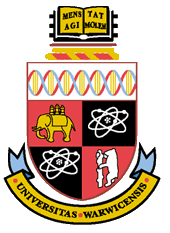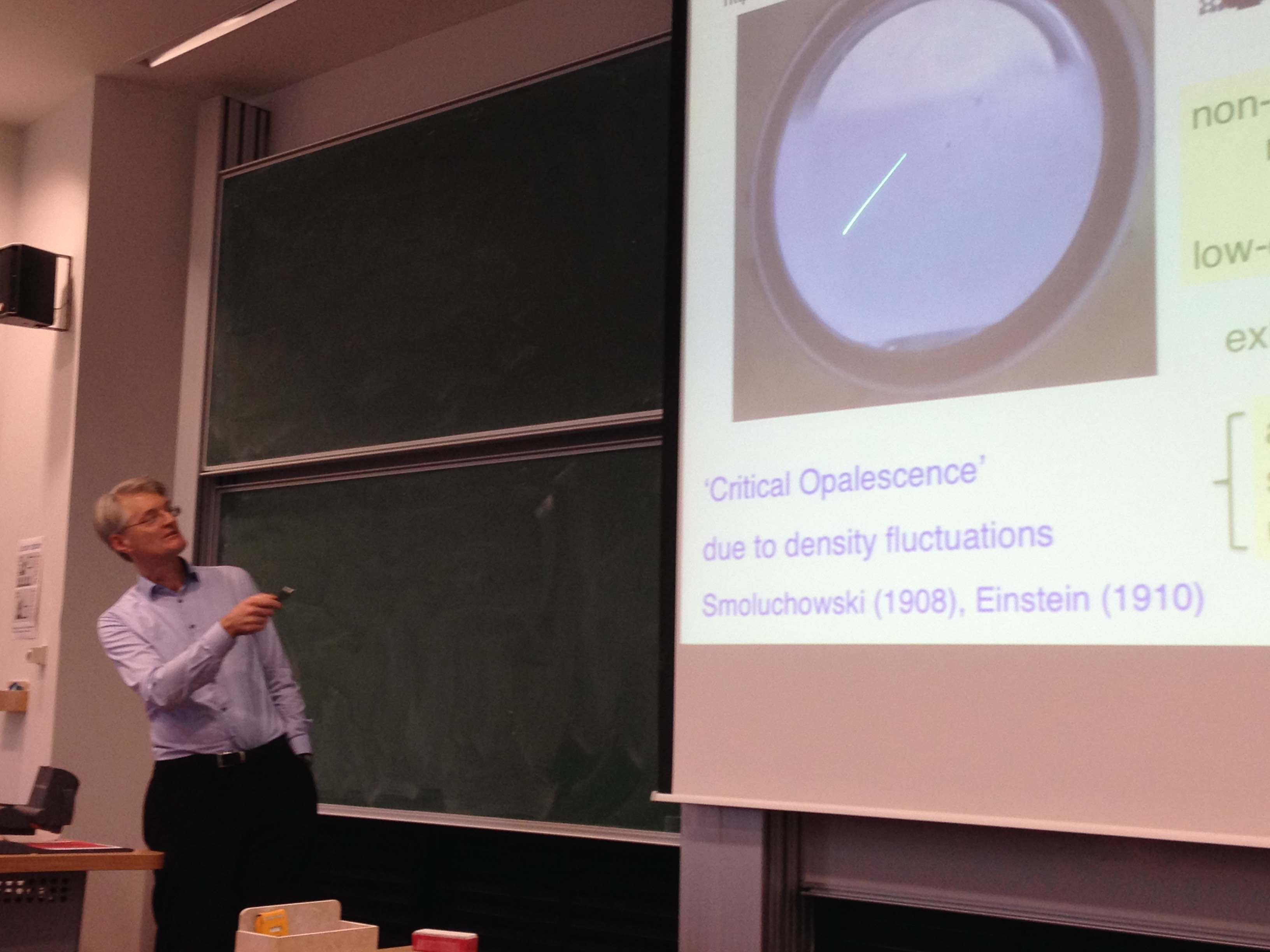#### This term, all seminars take place Thursdays at 2pm, room MS.04 (Zeeman Building), unless indicated otherwise.

Schedule forJeremy Allam, 13.11.2014

Seminars

 09.01.2020 Marielle Simon (Inria, Lille) CANCELLED 16.01.2020 Lorenzo Taggi (WIAS) Macroscopic self-avoiding walk interacting with lattice permutations and uniformly-positive monomer-correlations for the dimer model in Z^d, d > 2 This talk is about lattice permutations, namely permutations of the vertices of a box in Z^d such that every vertex is mapped to a nearest neighbour. Such objects can be viewed as ensembles of mutually-disjoint oriented self-avoiding loops and are sampled according to the uniform measure or to a measure which rewards the total number of loops. We consider a version of this model in which one of these loops is "open", namely is a self-avoiding walk. The motivation for studying lattice permutations when d > 2 is twofold: firstly, they are related to (loop representation of) the interacting quantum Bose gas, for which the existence of a 'regime with a long walk' is an important open conjecture. Secondly, the two-point function of lattice permutations equals the monomer-monomer correlation of the dimer model, a classical statistical mechanics model which is mostly unexplored when d > 2 (contrary to the two-dimensional dimer model, which is integrable and well-studied). We prove that the two-point function of lattice permutations is uniformly positive and that the "typical" distance of the end-points of the walk is of the same order of magnitude as the diameter of the box when d > 2. Our results follow from the derivation of a version of the so-called infrared bound from a new general probabilistic setting, with coloured loops and walks interacting at sites and walks entering into the system from some "virtual" vertices. 23.01.2020 Fabio Cunden (University College Dublin) Free fermions and α-determinantal processes Determinantal point processes were introduced in the 70s as a consistent description of non-interacting fermions in quantum mechanics. Determinantal processes arise naturally in several other settings, including eigenvalues of random matrices and nonintersecting paths. Another, perhaps not so well-known class of point processes are the so-called α-determinantal processes. Using the Gaussian case (harmonic oscillator) as paradigmatic example, I will illustrate a new limit procedure to construct α-determinantal processes out of excited states of free fermions. Joint work with Satya N. Majumdar and Neil O’Connell. 30.01.2020 Dirk Zeindler (University of Lancaster) Random permutations with logarithmic cycle weights The topic of this talk are random permutations on the symmetric group \$S_n\$ with logarithmically growing cycles weights. More precisely, we assign to each cycle in a given permutation the weight \$log^{k}(m)\$, where \$m\$ is the cycle length and \$kgeq 1\$ is an integer. We establish several limit theorems about the typical behaviour of a permutation under this measure as the length \$n\$ tends to \$infinity\$. We further compare the obtained results with the asymptotic behaviour under the uniform and the Ewens measure and also with random permutations with polynomial growing cycle weights. 06.02.2020 Jhih-Huang Li (University of Warwick) Phase transition in (1+1)-dimensional quantum random-cluster model The (1+1)-dimensional quantum random-cluster model can be seen as a 2D planar random-cluster model defined on a finer and finer rectangular lattice. In the talk, we start with definition and properties of the quantum model and its planar counterpart, discuss the link between them and give results on their phase transitions using known results from the square lattice. 13.02.2020 Benjamin Morris (University of Nottingham) The quantum Gibbs paradox This talk will be a pedagogical discussion of the Gibbs “paradox”. Starting from the classical solution based on the writings of Jaynes and ending with the presenter recent results on a quantum version that retains the paradoxes salient features, the paramount of which being the experimenter ability to resolve and address a system. In order to make the transition from a classical to a quantum description of said system we utilize foundation tools from representation theory that take into account both the uniquely identical nature of quantum particles and the experimenter ability to individually address them. This allows us to demonstrate not only a distinction between classical and quantum thermodynamics, but also a distinct thermodynamic advantage in the quantum case. We then push these results by finding the macroscopic limits of the setup to determine whether this quantum effect may be readily observed in experiment. 20.02.2020 Costanza Benassi (University of Northumbria) Thermodynamic Limit and Dispersive Regularisation in Matrix Models Random matrix models provide a universal paradigm for the modelling of complex phenomena. We focus on the Hermitian Matrix Model and its phase diagram. We show this model supports the occurrence of a novel type of phase transition characterised by dispersive regularisation of the order parameter near the critical point. Using the identification of the partition function with a solution of the integrable system known as Volterra lattice, we argue that the singularity is resolved via the onset of a multi-dimensional dispersive shock of the order parameter in the space of coupling constants. This analysis explains the origin and mechanism leading to the emergence of chaotic behaviours observed in M^6 matrix models and extends its validity to even nonlinearity of arbitrary order. Based on a joint work with A. Moro (arXiv:1903.11473). 27.02.2020 Robin Reuvers (University of Cambridge) A variational description of the weakly-interacting Bose gas Einstein classic treatment of Bose-Einstein condensation for non-interacting bosons predicts at what temperature the phase transition to BEC occurs, but how this critical temperature changes when the bosons interact weakly is an old problem, first posed by Feynman in 1953. In this talk, I will address this question in a variational model. I will also provide an introduction to the non-interacting case. 05.03.2020 Vincent Lerouvillois (Université de Lyon) Hydrodynamic limits for (2+1)-dimensional interface growth models in the anisotropic KPZ class Interface growth models are described microscopically by a random discrete height function that evolves according to an asymmetric Markovian dynamic (often related to interacting particles systems like ASEP or dimer models). The macroscopic behaviour of the interface is given by the Hydrodynamic limit (Law of Large Numbers) i.e the convergence of the space-time rescaled height function to the solution of a non-linear Hamilton-Jacobi PDE \$partial_t u = v( abla u)\$. Fluctuations are expected to share universal features in link with the KPZ equation, depending on the dimension and the convexity of \$v\$. I will present the Hydrodynamic limit of the Gates-Westcott model, a (2+1)-dimensional generalisation of the Polynuclear Growth Model in the anisotropic KPZ class 12.03.2020